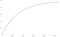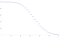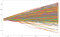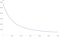# BITCOIN MINING CALCULATIONS

This is a writeup of Bitcoin mining related calculations useful to quantify mining profitability and its risks.

# The magnitude of required work

The minimum threshold for block validity is hardcoded in the Bitcoin source code, such that the expected number of hashes needed to find a solution is 2³². Current mining rigs perform 2⁴⁴ −2⁴⁵, the entire network ≈ 2⁶³ hashes in a second. The threshold of validity is regularly re-calibrated by the network such that it takes in average 10 minutes of joint search of all miners of the network to find a valid block. The difficulty of the current search is expressed as a factor of the hardcoded minimum difficulty. The expected work at a difficulty level d is d ∗ 2³² hashes.

# Get difficulty from the block chain

Mining difficulty is recorded in the block chain in every block, therefore it is its authoritative source. The encoding is rather specific and can be reverse engineered from Bitcoin source code as: interpret the 73rd byte of the block as a positive number x and 74–75th bytes as a big-endian positive number y. The block chain encoded difficulty is then:

The network re-adjusts mining difficulty such that it can be expected that the network finds a new solution in 10 minutes intervals. The adjustment takes place at every 2016th block.

# Network speed

Since a miner is expected to be successful in every 10 minutes, the implied total network computation speed is S(d) in hash/second is:

Using current example difficulty of 1590896927258, the implied network speed is: 11.38*10¹⁸ hash/s or 11.38 EH/s (exa hashes/s)

# Implied market share

Once network speed is known, a miner with can calculate its market share f from its own computation speed s and current difficulty d as:

# The Mining Reward

A miner is entitled to new Bitcoins with every block it produces and accepted by the network. The entitlement of new Bitcoins declines with the position of the new block on the chain: it started at 50 Bitcoins and halves every 210,000 blocks. The Bitcoin software uses integer arithmetic (only) and represents 1 Bitcoin as 10⁸ satoshis. The entitlement of new Bitcoins N for a given block height h is:

Due to implicit rounding-down in integer operations of the C++ language, N(h) drops to 0 for blocks with h >= 34 ∗ 210000. Since the network is calibrated to produce 1 blocks every 10 minutes, this is expected in 34∗210000/(6∗24)/365 ≈ 136 years after Bitcoin’s start in 2009.

Since this is the only source of new Bitcoins, their total quantity will never exceed 20999949.9769 ≈ 21 million Bitcoins. At the time point of this writing the number of Bitcoins in circulation is close to 17 million.

The miner receives in addition to new Bitcoins also the fees of transactions included into the block. The proportion of fees in miner’s income varies, currently around 15% and is expected to increase in future.

# Expected Bitcoin production

A miner with known hash speed s, that is a subset of the networks total, will have a proportionally longer expected time to solution than the network. Consequently the share of the network’s daily total reward will be proportional to market share as follows:

A 10 TH/s (10¹³ hash/s) miner at block height 57595 (H(57595) = 12.5) and current difficulty of 1590896927258, is expected produce at least 0.0015806 Bitcoins or 15860 bits today.

# Time to find a block

Performing hashes has no “progress”, means no matter how many calculations were performed earlier, the probability that the next hash calculated is the winner remains unchanged at 1/(d*2³²).

The time to next block for a hash speed s has therefore an exponential distribution with below cumulative density function (t is measured in seconds):Probability that a mining pool with 2.162 EH speed atdifficulty of 1590896927258 finds a block in number of seconds

# Mining pool production

The events of finding a block occur independently of the time since the last event, therefore the number of events within a time period follows a Poisson distribution. We can use the cumulative probability density function of the Poisson distribution to calculate the probability of finding more than k blocks in a day is:Probability that a pool with 2.162 EH/s at difficulty 1590896927258 finds more than given number of blocks a day.

# Modelling difficulty

It is seems that mining reward creates a feedback loop, that for most of Bitcoin’s history resulted in an acceleration of the network’s computing capacity.

The best fit model to historical data of monthly multiplicative difficulty changes is an autoregressive process:the parameters have strong t-statistics of 4.5 and 3.2 and the error variance is low 0.03Simulations of network speed in ExaHash/second for the next 12 months using above process.

Above observations allow us to assume change of difficulty continues the trend of the previous months. A simple model using a daily constant difficulty increase rate r should be sufficient for practical purposes over short time periods. We define expected difficulty for a future in t days as:

Previous simulation gives us an estimate r = 0.75% which corresponds to an annualized difficulty increase of 1429%.

# Expected Bitcoin income

The expected amount of Bitcoins a mining machine can produce within t days is determined by the current block reward and the difficulty rate increase. If we assume that there is no reward halving in the relevant lifetime, then N(h)=N, and the expected income is:1 year Bitcoin production of a 1 TH miner under assumption of difficulty increase rate

Independent, Bitcoin Developer since 2012, Former: CLA @ Digital Asset Holdings, VP @ CoinTerra, CEO @ Bits of Proof, Engineer, Financial Risk Manager.

## More from Tamas Blummer

Independent, Bitcoin Developer since 2012, Former: CLA @ Digital Asset Holdings, VP @ CoinTerra, CEO @ Bits of Proof, Engineer, Financial Risk Manager.